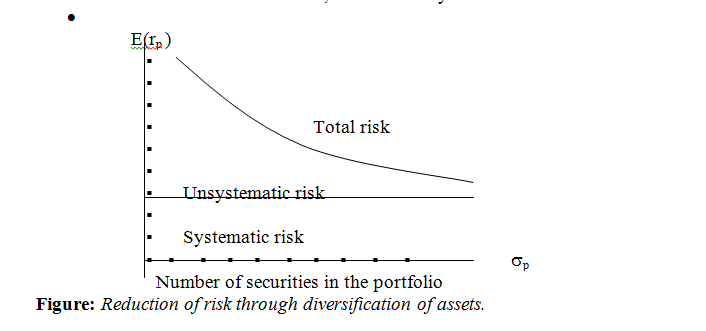Latest Articles
Home / Finance / Corporate Finance / Characteristics of Risk

# Characteristics of Risk

Characteristics of Risk.

The characteristics of risk can be summarized as below:

• Risk measures the changes in the product price.
• The more debt in the business, the riskier the investment.
• The larger the margin of safety, the lower the risk.
• The more variable the return, the riskier the investment.

Page Contents

# Characteristics of Risk## Return

Investment implies that investor defers current consumption in order to add to his/her wealth so that he/she can consume more in the future. So, the return on investment is concerned with the change in wealth resulting from the investment. Such a change in wealth can either be due to cash inflows in the form of interest or dividends or caused by a change in the price of the asset. The period during which an investor deploys funds (wealth) is termed as its holding period and the return for that period is called holding period return (HPR). HPR is calculated as:

 Ending value of the investment HPR = —————————————– Beginning value of the investment

## Holding Period Yield (HPY).

An investor can convert HPR to an annual percentage rate to derive a percentage return commonly known as holding period yield (HPY).

HPY is further be calculated as:

HPY = HPR−1

Again, the annual holding period return can be calculated as:

Annual HPR = [HPR]1/N

Where N is the number of years an investment holds. Suppose an investment is made by Tk. 200 the value of which would become art Tk.250 after 2 years.

The holding period return from the investment is:

HPR = [Ending value/ Beginning value]

= Tk.250/Tk.200 = 1.25

Annual HPR = [1.25]1/N = [1.25]1/2 = 1.1180

Therefore,

Annual HPY = 1.1180−1= 0.1180 =11.80%

If the investment is held for a period of 3 years, the holding period yield then would become:

Annual HPR = [1.25]1/N = [1.25]1/3 = 1.08

Therefore,

Annual HPY = 1.08 −1= 0.08 = 8%.

Alternatively, holding period return can be defined as the capital gains plus dividend per taka invested in the stock like:

HPR = [Capital gains + Dividend]/Investments

HPR = [(Ending price – Beginning price) + Cash dividends]/Beginning price

The ending value of the investment can be the result of a change in the price of the investment. Annual holding period yield assumes a constant annual yield for each year.

Another calculation of return is called geometric mean which is the nth root of the product of the holding period returns for n years. Geometric mean, therefore, is:

GM = [πHPR]1/N −1

Where,

π = the product of the annual holding period return calculated as:

[HPR1] × [HPR2] × ——- × [HPRn]

Suppose holding period returns for three consecutive years from an investment are 1.10, 1.15 and 1.20 respectively. The geometric mean return from the investment can be calculated as:

GM = [(1.10) × (1.15) × (1.20)] 1/3 − 1

= [1.518] 1/3 −1 = 1.1493−1= 0.1493 = 14.93%.